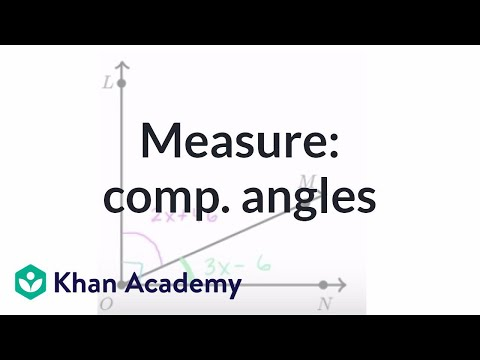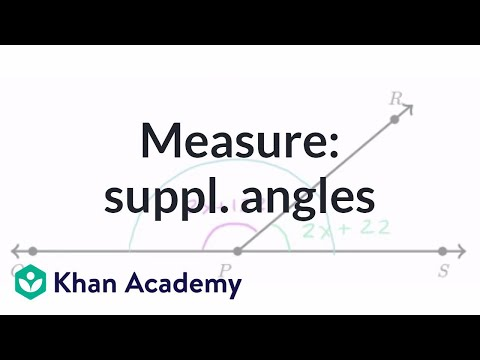Video

# Two-step equations with decimals and fractions (Full video)

Khan Academy

## Supporting grades: 7

Description: Learn how to solve equations that involve decimals and fractions. The equations shown in this video are called "two-step" equations because they each take two steps to solve. Let's get some practice solving equations that involve fractions and decimals. Since it's already on the right-hand side, let's try to get all the things that involve j on the right-hand side, and then get rid of everything else on the right-hand side. So I want to get rid of this negative 10/3, and the best way I can think of doing that is by adding 10/3.

You must log inorsign upif you want to:*

*Teacher Advisor is 100% free.

### Other videos you might be interested in### Equation practice with complementary angles (Full video)

#### Khan Academy